By Viraj Bhagat

Buying and selling options of the same type for the same stocks is called as Spread Trading. It limits your risk since you know the spread between the two options, but at the same time shrinks the reward, thus the Profits are limited. On creating a spread, the sale of an option offsets the purchase of an option. It offers visibility on risk Requires moderate to high level of trading, and thus for Advanced users

A bear spread is an option spread strategy opposite to that of a Bull Spread when the price of the underlying security is expected to fall. These strategies cost less to apply and are capped for Maximum Profit. Here, one Buys option and then sells an option of a lower strike price. If you are moderately bearish or want to reduce the cost of hedging your long positions, the bear call spread or bear put spread may be the answer.

### What Are Vertical Bear Spreads?

Vertical Spreads can be best explained as the following:

• Options with a: - lower striking price is sold - higher striking price is purchased
• Entering with a net credit or a net debit: Depends on whether calls or puts are used
• Gain and Loss has to be defined: Maximum loss and maximum gain for the trade is done by selecting strike prices of the options
• Objective: To profit when the stock price is expected to be steady or to go down (not significantly)

The qualities of a Bear Spread Strategy can be listed as follows:

• Used for excellent trades for when a stock is expected to go down but is likely to not go lower than a strong support level
• Profit or Max. Loss can be identified in advance
• Both spreads have a similar Payoff Structure
• Select strikes based on the time to expiry
• Profits and losses are capped

### When To Select A Bear Spread Strategy?

• The spread is best invoked when you are moderately bearish on the markets
• Implement the strategy only when you expect the volatility to increase (especially in the 2nd half of the series)
• If call option premiums are more attractive than put options - Choose bear call spread over a bear put spread
• If the stock collapses in the short term: Bear Put Spread is a better bet as the payout on long puts is immediate

Most Common Approach: If the trade goes opposite to the Trade, compare the maximum loss of each spread, identify which would generate the smallest loss and practise the CALL or the PUT spread accordingly

### What Do You Need For A Bear Spread Strategy?

The following criteria have to be met with if one wants to trade for Bear Spread Options:

• Underlying stock likely to fall in price
• Expiration date matching that of the stock price to fall
• Strike price

Consider: Price of  underlying asset/Stock that is believed to decrease in Price Risk: Simultaneously buying a higher striking ITM Put option and selling an OTM Put option at a lower strike price that reduces the cost, and thus the risk of the trade. Expiry of Options: Both options have the same expiry date

• Sell 1 OTM Put
• The only difference is the Strike Price

The strategy would ideally look something like this: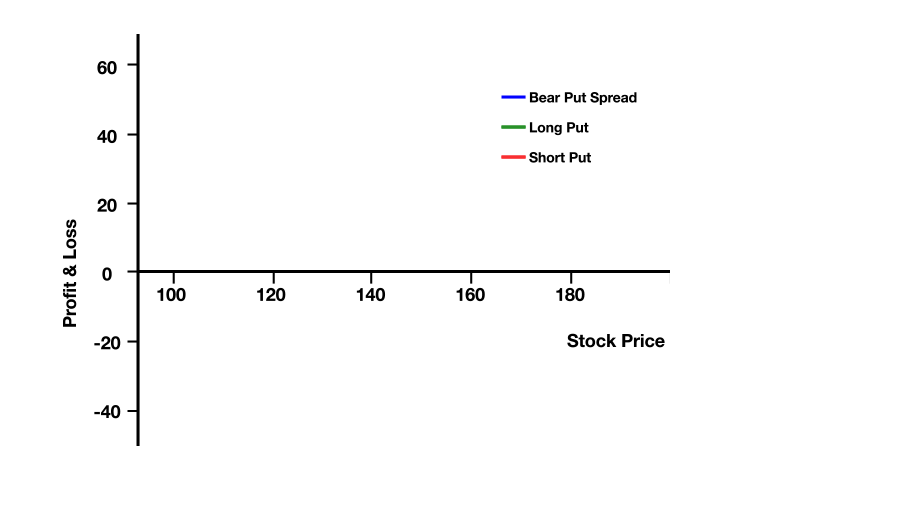Often, people seem to question the differences between Vertical Spreads for Put and Vertical Spreads for Call and different types of Bear Spreads by asking questions like:

Or

Or

Or

### What Are The Different Types Of Vertical Spreads?

Well, here is a brief comparison of both to help you understand the difference.

Established forA net credit if call options are usedA net debit if put options are used
Type of spreadCredit spread (results in net credit)Debit spread as a debit is taken upon entering the trade which results in net debit
Take this trade ifThe underlying stock is to remain below the strike price of the sold callThe underlying stock can go lower, but will not crash
CostLessMore
LeverageLessMore
ComplexityLessMore
Capital InvolvedMoreLess
BuyAt one strike price (OTM)At one strike price (ITM)
SellAt a lower strike price (ITM)At a lower strike price (OTM)
Money– Will immediately bring money into your account – You take in income at the outset of this trade– Will cost you money to buy it – You pay at the outset of the trade
LossesLimitedReduced
ProfitsLimited to the net premium received profit if the stock declines in valueLimited
Max. ProfitNet credit receivedDifference in the strike prices less the net cost of the trade
Max. RiskDifference in the strike prices less the net credit received at the outsetInitial cost of the spread
FormulaeNet Credit = Premium Received – Premium Paid Breakeven = Lower strike + Net Credit Max Profit = Net Credit Max Loss = Spread – Net CreditNet Debit = Premium Paid – Premium Received Breakeven = Higher strike – Net Debit Max Profit = Spread – Net Debit Max Loss = Net Debit
ObservedIn the final stages of a bear market or correction when stocks are nearing a troughNA
Strike PricesCall B > Call APut X > Put Y
Debit/CreditCreditDebit
When to use– If one is neutral or moderately bearish on a stock – The markets have rallied considerably (therefore CALL premiums have swelled) – The volatility is favorable – Ample time to expiryIf you are moderately bearish on a stock or other security

Now, to explain it in a simpler manner, utilising these concepts, I would take you through an example to gain a better understanding of the subject.

For this, we would take the example of Bear Put Options Trading Strategy Maximum Profit: Max Profit = Strike Price of Long Put - Strike Price of Short Put - Net Premium Paid Maximum Profit Potential = (Width of Put Strikes - Net Debit Paid) x 100 Price of Underlying </= Strike Price of Short Put Estimated Probability of Profit: Less than 50% for out-of-the-money spreads, approximately 50% for at-the-money spreads, and greater than 50% for in-the-money spreads. Maximum Loss: Max Loss = Net Premium Paid Maximum Loss Potential = Net Debit Paid x 100 Price of Underlying >/= Strike Price of Long Put Breakeven Point = Strike Price of Long Put - Net Premium Paid Expiration Breakeven Price = Long Put Strike Price - Net Debit Paid Resulting Position After Expiration: If the entire put spread is in-the-money at expiration, the long put expires to -100 shares and the short put expires to +100 shares, netting out to no stock position. However, if only the long put is in-the-money at expiration, the resulting position will be -100 shares per contract.

### Implementing The Bear Spread Options Strategy

I will use Adani Enterprises Ltd. (Ticker – NSE: ADANIENT) option for this example.

• Spot Price: 160
• Long Put Strike Price: 165 (Premium: 9.7)
• Short Put Strike Price: 155 (Premium: 5.4)

#### How To Calculate The Bear Spread Options Trading Strategy Payoff In Python?

Now, let me take you through the Payoff chart using the Python programming code.

##### Import Libraries
import numpy as np
import matplotlib.pyplot as plt
import seaborn
seaborn.set(style="darkgrid")

##### Put Payoff
def put_payoff(sT, strike_price, premium):
return np.where(sT < strike_price, strike_price - sT, 0) - premium

# Adani Enterprises Ltd Spot Price
s0 = 160

# Long Put
strike_price_long_put =165

# Short Put
strike_price_short_put = 155

# Range of put option at expiry
sT = np.arange(100,220,1)

fig, ax = plt.subplots()

ax.spines['bottom'].set_position('zero')
ax.plot(sT, long_put_payoff, color ='g')
ax.set_title('Long 165 Strike Put')
plt.xlabel('Stock Price (sT)')
plt.ylabel('Profit & Loss')
plt.show()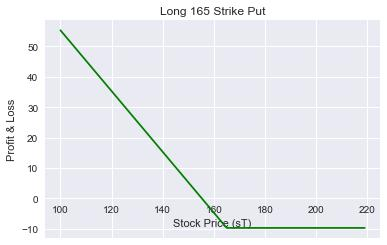short_put_payoff = put_payoff(sT, strike_price_short_put, premium_short_put) * -1.0

fig, ax = plt.subplots()
ax.spines['bottom'].set_position('zero')
ax.plot(sT, short_put_payoff, color ='r')
ax.set_title('Short 155 Strike Put')
plt.xlabel('Stock Price (sT)')
plt.ylabel('Profit & Loss')
plt.show()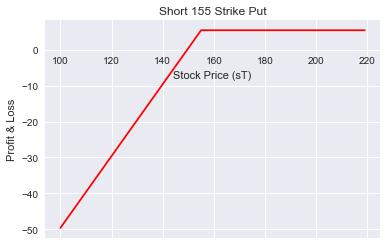##### Bear Put Payoff

The Payoff should ideally be as calculated in the tabular format here. You can download the excel sheet for calculating the Payoff of Bear Spread Strategy in the downloadable format at the end of the article.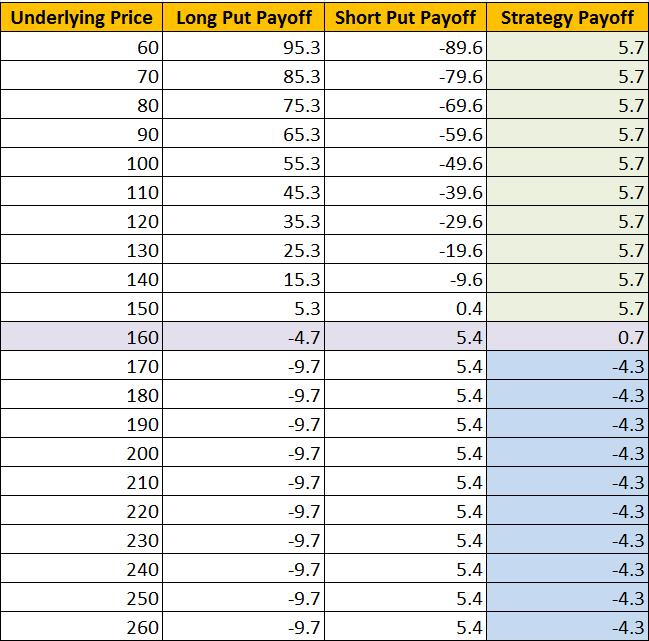fig, ax = plt.subplots(figsize=(10,5))
ax.spines['bottom'].set_position('zero')
ax.plot(sT, Bear_Put_payoff, color ='b', label = 'Bear Put Spread')
ax.plot(sT, long_put_payoff,'--', color ='g', label ='Long Put')
ax.plot(sT, short_put_payoff,'--', color ='r', label ='Short Put')
plt.legend()
plt.xlabel('Stock Price (sT)')
plt.ylabel('Profit & Loss')
plt.show()

The final output would look like this: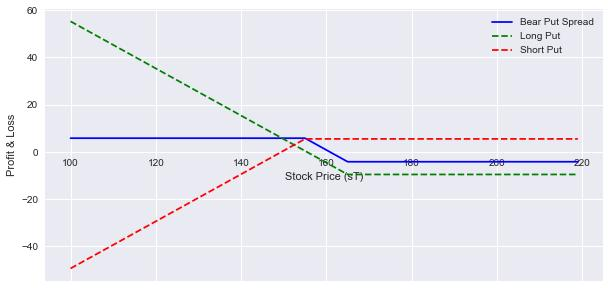Bear_Put_payoff = long_put_payoff + short_put_payoff

fig, ax = plt.subplots()
ax.spines['bottom'].set_position('zero')
ax.plot(sT, Bear_Put_payoff, color ='b')
plt.xlabel('Stock Price (sT)')
plt.ylabel('Profit & Loss')
plt.show()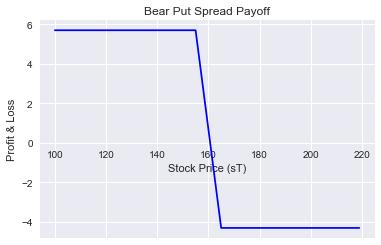profit = max (Bear_Put_payoff)
loss = min (Bear_Put_payoff)

print ("Max Profit %.2f" %profit)
print ("Max Loss %.2f" %loss)

5.70
-4.30

Max. Profit: 5.70 INR Max. Loss: -4.30 INR

### Conclusion

If you are modestly bearish, think volatility is rising and prefer to limit your risk, the best strategy would be a bear put spread.

Modern trading demands a systematic approach and the need to steer yourself away from trading from the gut. Learn how you too can trade options in a systematic manner with our course on Systematic Options Trading. Plus, you get to explore basics of options trading strategies like a butterfly, iron condor, and spread strategies. Enroll now!